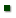Skip to main content
ARS Home » Research » Publications at this Location » Publication #166438

Title: PREDICTION OF METABOLIZABLE ENERGY REQUIREMENTS FOR MAINTENANCE AND GAIN OF PREWEANING, GROWING AND MATURE GOATS

AuthorLUO, JGOETSCH, ASAHLU, TNSAHLAI, IJOHNSON, ZMOORE, JGALYEAN, MOWENS, FFerrell, Calvin

 Submitted to: Small Ruminant Research Publication Type: Peer Reviewed Journal Publication Acceptance Date: 4/5/2004 Publication Date: 7/1/2004 Citation: Luo, J., Goetsch, A.L., Sahlu, T., Nsahlai, I.V., Johnson, Z.B., Moore, J.E., Galyean, M.L., Owens, F.N., Ferrell, C.L. 2004. Prediction of metabolizable energy requirements for maintenance and gain of preweaning, growing and mature goats. Small Ruminant Research. 53:231-252. Interpretive Summary: Treatment mean observations from published reports were used to construct databases to determine metabolizable energy for maintenance (MEm) and metabolizable energy for gain (MEg) requirements of goats by regressing metabolizable energy intake (MEI) against average daily gain (ADG). MEm was 485, 489, 580, 489 and 462 kJ/kg BW0.75, and MEg was 13.4, 23.1, 23.1, 19.8 and 28.5 kJ/g ADG for preweaning, growing meat, growing dairy, growing indigenous and mature goats, respectively. These estimates should be useful in diet formulation as well as prediction of performance of goats. However, goats in the reports used in this study were not subjected to appreciable stress, such as environmental or nutritional. Hence, application in some specific settings may require additional considerations. Technical Abstract: Databases were constructed to determine ME requirements for maintenance (MEm) and BW gain (MEg) of preweaning, growing and mature goats by regressing ME intake (MEI) against ADG. Goats were categorized as dairy, meat (>/_50% Boer) or indigenous biotypes. The preweaning database included 98 treatment means representing 1016 goats and the growing goat database consisted of 333 treatment means. Because of differences among biotypes of growing goats in intercepts and slopes (P < 0.05), separate regressions were performed. The meat subset included 60 observations from 11 publications, representing 548 goats; the dairy subset had 116 observations from 25 publications with 1851 goats; and the indigenous subset consisted of 157 observations from 34 publications and 1024 goats. Dairy and indigenous subsets were randomly split into independent sets for equation development and evaluation. The mature goat database included 69 treatment means from 23 publications and represented 495 goats. Small numbers of observations removed after initial regressions to improve fit did not markedly alter intercepts or slopes. Equations were as follows: preweaning: MEI (kJ/kg BW0.75) = 484.6 (S.E. = 61.46) + (13.37 [S.E. = 1.95] x ADG [g/kg BW0.75]) (n = 61; R2 = 0.44); meat: MEI (kJ/kg BW0.75) = 457.0 (S.E. = 22.30) + (25.23 [S.E. = 1.74] x ADG [g/kg BW0.75]) (n = 57; R2 = 0.79); dairy: MEI (kJ/kg BW0.75) = 573.7 (S.E. = 46.20) + (23.56 [S.E. = 3.10] x ADG [g/kg BW0.75]) (n = 56; R2 = 0.52); indigenous: MEI (kJ/kg BW0.75) = 500.0 (S.E. = 11.94) + (18.59 [S.E. = 1.64] x ADG [g/kg BW0.75]) (n = 76; R2 = 0.63); and mature: MEI (kJ/kg BW0.75) = 462.2 (S.E. = 24.95) + (28.52 (S.E. = 5.05) x ADG [g/kg BW0.75]) [n = 69; R2 = 0.32]. Intercepts and slopes from regressions of observed against predicted MEI with evaluation data sets, based on equations for preweaning and growing dairy and indigenous goats, were not different from 0 to 1, respectively. When final equations for the different growing goat biotypes were tested, the intercept for dairy goats differed (P < 0.05) from that of meat and indigenous goats, and the slope for indigenous goats tended (P = 0.16) to differ from that of meat and dairy goats. Therefore, the following dummy variable equation was obtained (I1 = 1 for dairy and 0 for others; I2 = 1 for indigenous and 0 for others): MEI (kJ/kg BW0.75) = 488.5 (S.E. = 14.4) + (91.5 (S.E. = 18.69) x I1) + (23.09 (S.E. = 1.24) x ADG [g/kg BW0.75]) ' (3.28 (S.E. = 1.98) x ADG [g/kg BW0.75] x I2) [n = 189; R2 = 0.74]. In summary, based on treatment mean observations from available publications and regression of MEI against ADG, MEm was 485, 489, 580, 489 and 462 kJ/kg BW0.75, and MEg was 13.4, 23.1, 23.1, 19.8 and 28.5 kJ/g ADG for preweaning, growing meat, growing dairy, growing indigenous and mature goats, respectively.